## Calculating Measurements of Basic Figures

Some basic figures, such as squares, rectangles, parallelograms, trapezoids, triangles, and circles, have measurements that are not difficult to calculate if the necessary information is given and the proper formula is used. You should first be familiar with the formulas of these basic figures.

#### Perimeter of some polygons—squares, rectangles, parallelograms, trapezoids, and triangles

Perimeter ( P) means the total distance all the way around the outside of the polygon (a many‐sided plane closed figure). The perimeter of that polygon can be determined by adding up the lengths of all the sides. The total distance around is the sum of all sides of the polygon. No special formulas are necessary, although the following two formulas are commonly seen:

• Perimeter ( P) of a square and a rhombus = 4 s ( s = length of side).
• Perimeter ( P) of a parallelogram and a rectangle = 2 l + 2 w or 2( l + w) ( l = length, w = width).

#### Area of polygons—squares, rectangles, parallelograms, trapezoids, and triangles

Area ( A) means the amount of space inside the polygon. Each type of polygon has a formula to determine its area.

A triangle is a three‐sided polygon. In a triangle, the base is the side the triangle is resting on, and the height is the distance from the base to the opposite point, or vertex.

Triangle: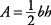( b = base, h = height). (See Figure 1.)

Figure 1 Triangles showing base and height.

##### Example 1

What is the area of the triangle shown in Figure 2?A square is a four‐sided polygon with all sides equal and all right angles (90 degrees). A rectangle is a four‐sided polygon with opposites sides equal and all right angles. In a square or rectangle, the bottom, or resting side, is the base, and either adjacent side is the height.

Square or rectangle: A = lw. (See Figure 3.)

Figure 2. Triangle showing base and height.

##### Example 2

What is the area of these polygons?

1. The square shown in Figure 4(a)

2. The rectangle shown in Figure 4(b)

1.2.A parallelogram is a four‐sided polygon with opposite sides parallel and equal. In a parallelogram, the resting side is usually considered the base, and a perpendicular line going from the base to the side opposite this base is the height.

Parallelogram: A = bh. (See Figure 5.)

Figure 4. Square and rectangle.Figure 5. Parallelogram showing base and height.##### Example 3

What is the area of the parallelogram shown in Figure 6?A trapezoid is a four‐sided polygon with only two sides parallel. In a trapezoid, the parallel sides are the bases, and the distance between the two bases is the height.

Trapezoid:. (See Figure 7.)

Figure 6. Parallelogram.Figure 7. Trapezoid showing bases and height.##### Example 4

What is the area of the trapezoid shown in Figure 8?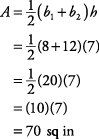What is the perimeter ( P) and the area ( A) of the polygons shown in Figure 9, (a) through (f), in which all measures are given in inches?

1.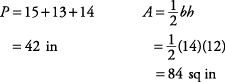2.3.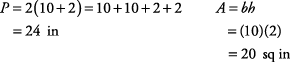4.5.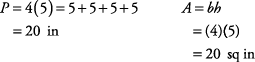6.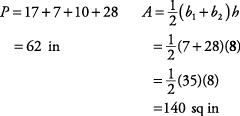#### Circumference and area of a circle

( C) is the distance around the circle. The diameter ( d) is the line segment that contains the center and has its end points on the circle. When the circumference of any circle is divided by its diameter, the result is always the same. That result is named after the Greek letter π (pi). The commonly used values for π are

π ≈ 3.14 or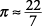Use either value in your calculations. The formula for circumference is

C = π d or C = 2π r

in which r = radius, a line segment from the center of the circle to one side, which is half the length of the diameter.

##### Example 6

is the circumference of the circle shown in Figure 10?

In the circle, r = 4, so d = 8.

C = πd

= π (8)

≈ 3.14(8) or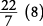25.12 in or ≈ 25.14 in

The area ( A) of a circle can be determined by

A = π r 2

##### Example 7

What is the area of the circle shown in Figure 11?

In the circle, d = 10, so r = 5.

A = π r 2

= π(5 2)

≈ 3.14(25) or78.5 sq in or ≈ 78.6 sq in

##### Example 8

From the given radius or diameter, find the area and circumference (leave in terms of π) of the circles in Figure 12.

1.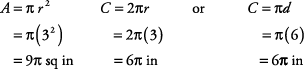2.Figure 12. Circles with dimensions.